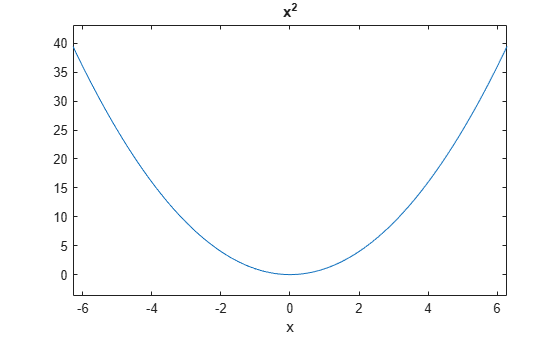# ezplot

(Not recommended) Easy-to-use function plotter

`ezplot` is not recommended. Use `fplot` instead. For more information, see Compatibility Considerations.

## Syntax

``ezplot(f)``
``ezplot(f,xinterval)``
``ezplot(f2)``
``ezplot(f2,xyinterval)``
``ezplot(funx,funy)``
``ezplot(funx,funy,uinterval)``
``ezplot(___,fig)``
``ezplot(ax,___)``
``h = ezplot(___)``

## Description

example

````ezplot(f)` plots the curve defined by the function `y = f(x)` over the default interval ```[-2π 2π]``` for `x`.`ezplot` automatically adds a title and axis labels to the plot.```
````ezplot(f,xinterval)` plots over the specified interval. Specify the interval as a two-element vector of the form `[xmin xmax]`.```
````ezplot(f2)` plots the curve defined by the implicit function `0 = f2(x,y)` over the default interval `[-2π 2π]` for `x` and `y`.```
````ezplot(f2,xyinterval)` plots over the specified interval. To use the same interval for both `x` and `y`, specify `xyinterval` as a two-element vector of the form `[min max]`. To use different intervals, specify a four-element vector of the form `[xmin xmax ymin ymax]`.```
````ezplot(funx,funy)` plots the parametrically defined planar curve defined by ```x = funx(u)``` and `y = funy(u)` over the default interval `[0 2π]` for `u`.```
````ezplot(funx,funy,uinterval)` plots over the specified interval. Specify the interval as a two-element vector of the form `[umin umax]`.```
````ezplot(___,fig)` plots into the specified figure window. Use any of the input argument combinations in the previous syntaxes that include an interval.```
````ezplot(ax,___)` plots into the axes specified by `ax` instead of the current axes `gca`. Specify the axes before any of the input argument combinations in any of the previous syntaxes except for the ones that involve `fig`. This syntax does not support the `fig` input.```
````h = ezplot(___)` returns either a chart line or contour object. Use `h` to modify the chart line or contour after it is created. For a list of properties, see Line Properties and Contour Properties.```

## Examples

collapse all

Plot the explicit function ${x}^{2}$ over the domain $\left[-2\pi ,2\pi \right]$.

`ezplot('x^2')`The default domain is $\left[-2\pi ,2\pi \right]$.

## Input Arguments

collapse all

Function to plot, specified as a character vector, string scalar, or function handle to a named or anonymous function.

Specify a function of the form `y = f(x)`. The function must accept a vector input argument and return a vector output argument of the same size.

When specifying the function as a character vector or string scalar, array multiplication, division, and exponentiation are always implied. For example, `x^2` is interpreted as `x.^2`.

Example: `'x^2'`

When specifying the function as a function handle, use array operators instead of matrix operators for the best performance. For example, use `.*` (`times`) instead of * (`mtimes`).

Example: `@(x) sin(x).*cos(x)`

Interval for `x`, specified as a two-element vector of the form `[xmin xmax]`.

Implicit function to plot, specified as a character vector, string scalar, or function handle to a named or anonymous function.

Specify a function of the form `0 = f(x,y)`. The function must accept two vector input arguments and return a vector output argument of the same size.

When specifying the function as a character vector or string scalar, array multiplication, division, and exponentiation are always implied. For example, `x^2` is interpreted as `x.^2`.

Example: `'x^2 - y^4'`

When specifying the function as a function handle, use array operators instead of matrix operators for the best performance. For example, use `.*` (`times`) instead of * (`mtimes`).

Example: `@(x,y) x.^2 - y.^2 - 1`

Plotting interval for `x` and `y`, specified in one of these forms:

• Vector of form `[min max]` — Use the interval `[min max]` for both `x` and `y`.

• Vector of form `[xmin xmax ymin ymax]` — Use the interval `[xmin xmax]` for `x` and `[ymin ymax]` for `y`.

Parametric function for x coordinates, specified as a function handle to a named or anonymous function.

Specify a function of the form `x = funx(u)`. The function must accept a vector input argument and return a vector output argument of the same size.

When specifying the function as a character vector or string scalar, array multiplication, division, and exponentiation are always implied. For example, `x^2` is interpreted as `x.^2`.

Example: `'sin(4*u)'`

When specifying the function as a function handle, use array operators instead of matrix operators for the best performance. For example, use `.*` (`times`) instead of * (`mtimes`).

Example: `@(u) sin(2.*u)`

Parametric function for y coordinates, specified as a function handle to a named or anonymous function.

Specify a function of the form `y = funy(u)`. The function must accept a vector input argument and return a vector output argument of the same size.

When specifying the function as a character vector or string scalar, array multiplication, division, and exponentiation are always implied. For example, `x^2` is interpreted as `x.^2`.

Example: `'cos(u*2)'`

When specifying the function as a function handle, use array operators instead of matrix operators for the best performance. For example, use `.*` (`times`) instead of * (`mtimes`).

Example: `@(u) -u.*cos(u)`

Plotting interval for `u`, specified as a two-element vector of the form `[umin umax]`.

Axes object. If you do not specify an axes object, then `ezplot` uses the current axes (`gca`).

## Version History

Introduced before R2006a

expand all